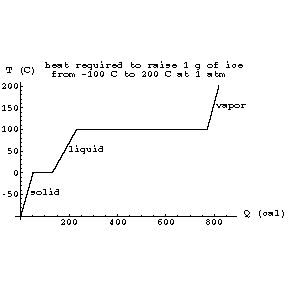# Heat

Heat (Q) is "thermal" energy: the energy of a statistical system of particles randomly colliding with each other and objects in their environment. It has dimensions of energy, but it is NOT a state variable: unlike temperature, its value DOES depend on the past history of the system. For instance, a system can be isothermally expanded by adding heat, or its pressure can be slowly decreased without the addition of heat. Yet the final pressure, temperature and volume are the same.

The heat energy of a system can be written as the product of two state variables, temperature and "entropy" (denoted S). Entropy is a measure of the "degeneracy" of a system (the number of states which all have the same energy, and are therefore all equally accessible):

S = k ln (n),

where n is the degeneracy. For example, all of the billiard balls on a table have the same gravitational potential energy; since there are a very large number of possible arrangements on the table, their entropy is high. When they are racked, the number of possible arrangements drops dramatically, and so too does their entropy. Note that in discussing only the balls, we have ignored the agent of their decrease in entropy: the billiard player! Her increase in entropy (as we will see in section E) more than compensated for the decrease of the balls'.

Entropy is an intrinsic state variable. In a completely isolated system, it can never decrease. Since high degeneracy is associated with randomness, it is often said that entropy is a measure of the "disorder" of a system. Disorder is a convenient concept for desktops and closets, but is too imprecise for physics. We will therefore forget about it when we make use of entropy in Sections E and F.

Heat is most often measured in "calories" (cal). A calorie is 4.186 J; it is the amount of heat needed to raise one gram of water 1K. A dietary "Calorie" (Cal) is 1000 calories, and we distinguish between the two by the capitalization.

The temperature of a substance changes as heat energy is added to it. The "heat capacity" (C) of an object is the ratio of change in heat to change in temperature, and the "specific heat" (c) of a substance is the heat capacity per unit mass. We therefore have

DQ = m c DT.

The specific heat of water is 1 cal / g K by definition. That of ice is .51 and of water vapor is .48 (at constant pressure). Ice and water vapor (steam) are alternate "phases" of water. The specific heat of human tissue is .85. That of air is .23.

For a given substance at a given pressure, phase changes occur at well-defined temperatures. For water at standard atmospheric pressure (at the surface of the earth), those are 273.15K and 373.15K (0 C and 100C, the freezing and boiling points, which define the Celsius degree, and therefore the Kelvin). For a given substance, the heat change per unit mass required for a phase transition is called the "latent heat" (of either "fusion" or vaporization) L. This means that

DQ = m L.

The latent heat of fusion (freezing) of water is 80 cal / g, and the latent heat of vaporization of water is 540 cal / g at 100 C. Note that the heat added or lost during a phase change does not affect the temperature during the phase change. Icewater is at 0 C until all of the water has frozen; when melting, it is at 0 C until all of the ice has melted. Likewise, water is at 100 C until all the water has boiled away; if the water / steam system is in a closed environment, the steam is at 100 C until the water has all evaporated. Hence we can graph the temperature vs heat of a substance:The horizontal portions of the graph represent the phase changes.

The next section is about body temperature regulation.

If you have stumbled on this page, and the equations look funny (or you just want to know where you are!), see the College Physics for Students of Biology and Chemistry home page.